Retour sur la compétition Kaggle “Human or Robot?”Cet article n’est pas encore disponible en français. Toutes mes excuses.

A month ago, I started participating in my first Kaggle competition. I wanted to start participating in Kaggle competitions for a while and Facebook launched a recruiting competition: Human or Robot?, and I decided to join the party.

I was finally ranked 32nd on the final ranking (private learderboard), and I could actually have been ranked 17th if I had chosen another of my predictions as final submission… (That additionnal URL feature wasn’t that useless afterall…)

This blog post is a (slightly modified) export of my IPython notebooks written for this competition. Well… Let’s get started!

TL;DR: I used a bunch of basic statistical features extracted from aggregation of bids of each bidder and trained a Random Forest tuned by 10-fold-CV. Plain and simple.

Import needed libraries for dataset creation

Nothing fancy here, we just use some classical Python Data Science libraries: numpy, scikit-learn and pandas, plus pickle to save the result.

import pickle
import numpy as np
import sklearn.preprocessing
from sklearn_pandas import DataFrameMapper
import pandas as pd


First step is to read the CSV files and load them as Pandas frames. I used Pandas here because of the « heavy » work needed to create the features, being very easy to do with Pandas and that would have been much more painful to do with numpy only for example.

# Read the bids, replace NaN values with a dash because NaN are string categories we don't know
bids.fillna('-', inplace=True)

# Load train and test bidders lists (nothing really interesting in that list)

# Join those 2 datasets together (-1 outcome meaning unknown i.e. test)
test['outcome'] = -1.0
bidders = pd.concat((train, test))


Dataset investigation

Prior to the feature creation, some inspection on the data has been done, of course, but I didn’t kept any trace of this quick’n’dirty work anywhere and won’t be able to show it to you.

The idea is to get to know what’s in the dataset. The first step is just to show look at the raw data, and to then look at some stats about the data. For example, the first thing you might wonder is what the heck can I do with those payment_account and address hashes that Kaggle gave me for each bidder. Is there any of these things that appear more than once? Nope, they are as unique as the bidder_id so you can throw this away. At this moment you know that all your features must come from the bids themselves.

Then you do some stats and plots of what’s in the bids, you look what could be interesting to compute features…

Features creation

… and then there is a moment when you decide that it’s time to get this party started and start to do something with these bids.

The things I hesitated about was to decide if I should predict if a bidder is a bot based on aggregates of info about his bids, or if I should predict if a bid has been made by a bot for each bid and then aggregate the predictions at bid level to create a prediction at bidder level.

I preferred to work at the bidder level because I had the feeling that each bid don’t have enough info by itself to allow proper prediction, and that the aggregation of a set of bids would allow me to create more high level features about the general behavior of a bidder and therefore get more useful info.

I didn’t have the time to actually try the bid-level prediction approach. I don’t know how it would have turned out.

So the first thing you do is to look at the info you have on each bid and look at what feature you can compute with this. We have this:

• auction (category) – Unique identifier of an auction
• merchandise (category) – The category of the auction site campaign, which means the bidder might come to this site by way of searching for « home goods » but ended up bidding for « sporting goods » – and that leads to this field being « home goods ». This categorical field could be a search term, or online advertisement.
• device (category) – Phone model of a visitor
• time (real) – Time that the bid is made (transformed to protect privacy).
• country (category) – The country that the IP belongs to
• ip (category) – IP address of a bidder (obfuscated to protect privacy).
• url (category) – url where the bidder was referred from (obfuscated to protect privacy).

We only have one real feature and lots of categories.

My first approach was what I’ve seen called « kitchen sink approach », I basically decided to compute whatever statistical computation crossed my mind (as long as I wasn’t too lazy to implement it so it had better be simple or genius). I decided to apply the same analysis on all categories. And the same idea goes for the real variable, with different stats of course.

Category variable feature extraction

The idea is to group the bids of a bidder and compute stats about the group (which is therefore a series of value for each variable). Then for a list of string, you get stats about these strings and their frequencies:

• number of unique categories that appear
• highest frequency that appearance
• lowest frequency of appearance
• category that appear the most
• standard deviation of the frequencies

and… that’s it!

def computeStatsCat(series, normalizeCount = 1.0):

n = float(series.shape)
counts = series.value_counts()

nbUnique = counts.count() / normalizeCount
hiFreq = counts / n
loFreq = counts[-1] / n
argmax = counts.index
stdFreq = np.std(counts / n)

return (nbUnique, loFreq, hiFreq, stdFreq, argmax)


Real variable feature extraction

For time, I decided to go a little bit deeper and group the series of timestamp of bids by auction and have two stages of stats, stats at auction level that are then aggregated and global stats for the whole set of timestamps of the bidder.

You see below a few functions that allowed me to compute those features. Basically, for each auction I compute stats that are: min, max, range of timestamps, and then I compute a bunch of things about interval between two bids: mean interval, standard deviation, percentiles. I then aggregate those results for all the auction a bidder had always in the same ideas of simple stats.

# Compute stats of numerical series without caring about interval between values
def computeStatsNumNoIntervals(series):

min = series.min()
max = series.max()
mean = np.mean(series)
std = np.std(series)
perc20 = np.percentile(series, 20)
perc50 = np.percentile(series, 50)
perc80 = np.percentile(series, 80)

return (min, max, mean, std, perc20, perc50, perc80)

# Compute stats of a numerical series, taking intervals between values into account
def computeStatsNum(series, copy = True):

if copy:
series = series.copy()

series.sort()
intervals = series[1:].as_matrix() - series[:-1].as_matrix()
if len(intervals) < 1:
intervals = np.array()

nb = series.shape
min = series.min()
max = series.max()
range = max - min
intervalsMin = np.min(intervals)
intervalsMax = np.max(intervals)
intervalsMean = np.mean(intervals)
intervalsStd = np.std(intervals)
intervals25 = np.percentile(intervals, 25)
intervals50 = np.percentile(intervals, 50)
intervals75 = np.percentile(intervals, 75)

return (nb, min, max, range,
intervalsMin, intervalsMax, intervalsMean, intervalsStd,
intervals25, intervals50, intervals75)

# Compute stats about a numerical column of table, with stats on sub-groups of this column (auctions in our case).
def computeStatsNumWithGroupBy(table, column, groupby):

# get series and groups
series = table[column]
groups = table.groupby(groupby)

# global stats
(nb, min, max, range,
intervalsMin, intervalsMax, intervalsMean, intervalsStd,
intervals25, intervals50, intervals75) = computeStatsNum(series)

# stats by group
X = []
for _, group in groups:
(grpNb, _, _, grpRange, grpIntervalsMin, grpIntervalsMax, grpIntervalsMean, grpIntervalsStd, _, _, _) = computeStatsNum(group[column])
X.append([grpNb, grpRange, grpIntervalsMin, grpIntervalsMax, grpIntervalsMean, grpIntervalsStd])
X = np.array(X)

grpNbMean = np.mean(X[:,0])
grpNbStd = np.std(X[:,0])
grpRangeMean = np.mean(X[:,1])
grpRangeStd = np.std(X[:,1])
grpIntervalsMinMin = np.min(X[:,2])
grpIntervalsMinMean = np.mean(X[:,2])
grpIntervalsMaxMax = np.max(X[:,3])
grpIntervalsMaxMean = np.mean(X[:,3])
grpIntervalsMean = np.mean(X[:,4])
grpIntervalsMeanStd = np.std(X[:,4])
grpIntervalsStd = np.mean(X[:,5])

return (nb, min, max, range,
intervalsMin, intervalsMax, intervalsMean, intervalsStd,
intervals25, intervals50, intervals75,
grpNbMean, grpNbStd, grpRangeMean, grpRangeStd,
grpIntervalsMinMin, grpIntervalsMinMean, grpIntervalsMaxMax, grpIntervalsMaxMean,
grpIntervalsMean, grpIntervalsMeanStd, grpIntervalsStd)


Feature tried that did not really worked

From categories to real values

In a desperate attempt to increase my score, I though about replacing categories in the bids dataset by real values by computing general stats about each category of each variable, and replacing this category by stats about this category, in my case the probability of this category to belong to appear in a bot’s bid.

I am aware that this is getting close to the danger of Data Leakage because you are explicitly introducing information about the target in the features. However, I feel like because the real value you use to represent the category is computed on the whole dataset, it might in some cases be ok because it is a very aggregated info, provided you have a lot of data in each category.

In this case, I think that if was definitely a data leakage because I got a 0.97 AUC on my CV but a 0.86 score on public leaderboard (a big drop from my results without those features). But it was worth trying!

def computeOutcomeProbaByCat(data, cats):
stats = {}
for cat in cats:
stats[cat] = pd.DataFrame(data.groupby(cat).aggregate(np.mean).outcome)
stats[cat].rename(columns={'outcome': cat+'Num'}, inplace=True)
return stats

#bidsWithOutcome = pd.merge(bids, bidders[['outcome']], how='left', left_on='bidder_id', right_index=True)
#stats = computeOutcomeProbaByCat(bidsWithOutcome[bidsWithOutcome.outcome >= 0], [u'auction', u'merchandise', u'device', u'country', u'url'])

# Add real columns to bids dataframe
#for cat in stats:
#    bids = pd.merge(bids, stats[cat], how='left', left_on=cat, right_index=True)


Make a special case for merchandise category

I also wanted to make a special case for merchandise category, and have a couple of features per category indicating in a way how the bidder participated in the auctions of this merchandise: the number of bids in the category and the percentage of his bids made in this category.

This did not changed my score in any way, probably due to the fact that very few bidders actually participate in multiple merchandises if I remember well.

Features I didn’t tried

There are a lot of features I could have tried if I had time and motivation. You will find a lot of different things in others feedback from this contest. There are a lot of great and nice features I do not have, however, it seems that what I got here already gives you pretty good results.

Lots of people like to look at the contribution of each feature in the final classifiers. I’m sure it might give some information and ideas about how to improve your features. I didn’t do it in this competition. And I’m anyway not a big fan of interpreting a Machine Learning model, something also « criticized » in the great kdnuggets blog article « The Myth of Model Interpretability ».

Global computation of features

Well, finally we need to compute all those features from our dataset so, I know this block of code is kind of dirty, but since I wanted to be able to include of exclude features at ease, this was my solution. This is the moment when multi-cursors feature of Sublime Text takes stats being very useful.

# Init vars
Xids = []
X = []

# merchandises = bids.merchandise.value_counts()

# For each bidder
for bidder, group in bids.groupby('bidder_id'):

# Compute the stats
(nbUniqueIP, loFreqIP, hiFreqIP, stdFreqIP, IP) = computeStatsCat(group.ip)
(nbUniqueDevice, loFreqDevice, hiFreqDevice, stdFreqDevice, device) = computeStatsCat(group.device)
(nbUniqueMerch, loFreqMerch, hiFreqMerch, stdFreqMerch, merch) = computeStatsCat(group.merchandise)
(nbUniqueCountry, loFreqCountry, hiFreqCountry, stdFreqCountry, country) = computeStatsCat(group.country)
(nbUniqueUrl, loFreqUrl, hiFreqUrl, stdFreqUrl, url) = computeStatsCat(group.url)
(nbUniqueAuction, loFreqAuction, hiFreqAuction, stdFreqAuction, auction) = computeStatsCat(group.auction)
(auctionNb, auctionMin, auctionMax, auctionRange,
auctionIntervalsMin, auctionIntervalsMax, auctionIntervalsMean, auctionIntervalsStd,
auctionIntervals25, auctionIntervals50, auctionIntervals75,
auctionGrpNbMean, auctionGrpNbStd, auctionGrpRangeMean, auctionGrpRangeStd,
auctionGrpIntervalsMinMin, auctionGrpIntervalsMinMean, auctionGrpIntervalsMaxMax, auctionGrpIntervalsMaxMean,
auctionGrpIntervalsMean, auctionGrpIntervalsMeanStd, auctionGrpIntervalsStd) = computeStatsNumWithGroupBy(group, 'time', 'auction')

# Save the stats
# Also I don't really remember which category features I kept or not in my final submission :# I think it was IP + device + merch + contry, but for computation time let's comment some of these x = [nbUniqueIP, loFreqIP, hiFreqIP, stdFreqIP, #IP, nbUniqueDevice, loFreqDevice, hiFreqDevice, stdFreqDevice, #device, nbUniqueMerch, loFreqMerch, hiFreqMerch, stdFreqMerch, merch, nbUniqueCountry, loFreqCountry, hiFreqCountry, stdFreqCountry, country, nbUniqueUrl, loFreqUrl, hiFreqUrl, stdFreqUrl, #url, nbUniqueAuction, loFreqAuction, hiFreqAuction, stdFreqAuction, #auction auctionNb, auctionMin, auctionMax, auctionRange, auctionIntervalsMin, auctionIntervalsMax, auctionIntervalsMean, auctionIntervalsStd, auctionIntervals25, auctionIntervals50, auctionIntervals75, auctionGrpNbMean, auctionGrpNbStd, auctionGrpRangeMean, auctionGrpRangeStd, auctionGrpIntervalsMinMin, auctionGrpIntervalsMinMean, auctionGrpIntervalsMaxMax, auctionGrpIntervalsMaxMean, auctionGrpIntervalsMean, auctionGrpIntervalsMeanStd, auctionGrpIntervalsStd] ## Old stats per merchandise # for key in merchandisesCounts.index: # merchandisesTmp[key] = merchandisesCounts[key] # merchandisesTmp2[key] = float(merchandisesCounts[key]) / len(group) # merchandisesTmp = merchandises * 0 # merchandisesTmp2 = (merchandises * 0).astype('float') # merchandisesCounts = group.merchandise.value_counts() # x += merchandisesTmp.tolist(); # x += merchandisesTmp2.tolist(); # Old stats replacing using real value substitution of categories # catCols = [] # for cat in stats: # (catMin, catMax, catMean, catStd, catPerc20, catPerc50, catPerc80) = computeStatsNumNoIntervals(group[cat+'Num']) # x += [catMin, catMax, catMean, catStd, catPerc20, catPerc50, catPerc80] # Save the stats in the result arrays Xids.append(bidder) X.append(x) # Features labels Xcols = ['nbUniqueIP', 'loFreqIP', 'hiFreqIP', 'stdFreqIP', #'IP', 'nbUniqueDevice', 'loFreqDevice', 'hiFreqDevice', 'stdFreqDevice', #'device', 'nbUniqueMerch', 'loFreqMerch', 'hiFreqMerch', 'stdFreqMerch', 'merch', 'nbUniqueCountry', 'loFreqCountry', 'hiFreqCountry', 'stdFreqCountry', 'country', 'nbUniqueUrl', 'loFreqUrl', 'hiFreqUrl', 'stdFreqUrl', #'url', 'nbUniqueAuction', 'loFreqAuction', 'hiFreqAuction', 'stdFreqAuction','auctionNb', 'auctionMin', 'auctionMax', 'auctionRange', 'auctionIntervalsMin', 'auctionIntervalsMax', 'auctionIntervalsMean', 'auctionIntervalsStd', 'auctionIntervals25', 'auctionIntervals50', 'auctionIntervals75', 'auctionGrpNbMean', 'auctionGrpNbStd', 'auctionGrpRangeMean', 'auctionGrpRangeStd', 'auctionGrpIntervalsMinMin', 'auctionGrpIntervalsMinMean', 'auctionGrpIntervalsMaxMax', 'auctionGrpIntervalsMaxMean', 'auctionGrpIntervalsMean', 'auctionGrpIntervalsMeanStd', 'auctionGrpIntervalsStd'] # Old features labels when replacing using real value substitution of categories # for cat in stats: # Xcols += [cat + 'NumMin', cat + 'NumMax', cat + 'NumMean', cat + 'NumStd', cat + 'NumPerc20', cat + 'NumPerc50', cat + 'NumPerc80'] # Xcols += map(lambda x: "merch" + x + "Abs", merchandisesTmp.keys().tolist()) # Xcols += map(lambda x: "merch" + x + "Prop", merchandisesTmp.keys().tolist()) # Create a pandas dataset, remove NaN from dataset and show it dataset = pd.DataFrame(X,index=Xids, columns=Xcols) dataset.fillna(0.0, inplace=True) dataset  nbUniqueIP loFreqIP hiFreqIP stdFreqIP nbUniqueDevice loFreqDevice hiFreqDevice stdFreqDevice nbUniqueMerch loFreqMerch auctionGrpNbStd auctionGrpRangeMean auctionGrpRangeStd auctionGrpIntervalsMinMin auctionGrpIntervalsMinMean auctionGrpIntervalsMaxMax auctionGrpIntervalsMaxMean auctionGrpIntervalsMean auctionGrpIntervalsMeanStd auctionGrpIntervalsStd 001068c415025a009fee375a12cff4fcnht8y 1 1.000000 1.000000 0.000000e+00 1 1.000000 1.000000 0.000000e+00 1 1 0.000000 0.000000e+00 0.000000e+00 0 0.000000e+00 0.000000e+00 0.000000e+00 0.000000e+00 0.000000e+00 0.000000e+00 002d229ffb247009810828f648afc2ef593rb 1 1.000000 1.000000 0.000000e+00 2 0.500000 0.500000 0.000000e+00 1 1 0.000000 1.052632e+08 0.000000e+00 105263158 1.052632e+08 1.052632e+08 1.052632e+08 1.052632e+08 0.000000e+00 0.000000e+00 0030a2dd87ad2733e0873062e4f83954mkj86 1 1.000000 1.000000 0.000000e+00 1 1.000000 1.000000 0.000000e+00 1 1 0.000000 0.000000e+00 0.000000e+00 0 0.000000e+00 0.000000e+00 0.000000e+00 0.000000e+00 0.000000e+00 0.000000e+00 003180b29c6a5f8f1d84a6b7b6f7be57tjj1o 3 0.333333 0.333333 0.000000e+00 3 0.333333 0.333333 0.000000e+00 1 1 0.000000 0.000000e+00 0.000000e+00 0 0.000000e+00 0.000000e+00 0.000000e+00 0.000000e+00 0.000000e+00 0.000000e+00 00486a11dff552c4bd7696265724ff81yeo9v 10 0.050000 0.300000 7.071068e-02 8 0.050000 0.350000 1.060660e-01 1 1 0.634324 1.518721e+12 2.301461e+12 0 1.090126e+12 5.571737e+12 1.401996e+12 1.246061e+12 1.775155e+12 1.559352e+11 ffbc0fdfbf19a8a9116b68714138f2902cc13 18726 0.000040 0.006341 1.099251e-04 792 0.000040 0.096989 4.973862e-03 1 1 197.065280 5.318327e+12 4.887044e+12 0 4.775914e+11 1.243363e+13 2.360377e+12 9.546782e+11 1.483847e+12 6.113005e+11 ffc4e2dd2cc08249f299cab46ecbfacfobmr3 18 0.045455 0.090909 1.889726e-02 13 0.045455 0.181818 4.504930e-02 1 1 0.805536 9.881656e+12 2.341891e+13 0 5.617653e+12 7.443268e+13 9.329175e+12 7.038926e+12 1.881787e+13 1.635072e+12 ffd29eb307a4c54610dd2d3d212bf3bagmmpl 1 1.000000 1.000000 0.000000e+00 1 1.000000 1.000000 0.000000e+00 1 1 0.000000 0.000000e+00 0.000000e+00 0 0.000000e+00 0.000000e+00 0.000000e+00 0.000000e+00 0.000000e+00 0.000000e+00 ffd62646d600b759a985d45918bd6f0431vmz 37 0.001506 0.055723 1.672626e-02 96 0.001506 0.301205 3.164567e-02 1 1 17.389500 4.444518e+12 4.925281e+12 0 1.002909e+11 9.788421e+12 1.723225e+12 5.569457e+11 7.713609e+11 5.499901e+11 fff2c070d8200e0a09150bd81452ce29ngcnv 1 1.000000 1.000000 0.000000e+00 1 1.000000 1.000000 0.000000e+00 1 1 0.000000 0.000000e+00 0.000000e+00 0 0.000000e+00 0.000000e+00 0.000000e+00 0.000000e+00 0.000000e+00 0.000000e+00 6614 rows × 48 columns Saving the final dataset Now that we have a nice dataset, we need to make it a Machine Learnable one. Because we still have categories, we have variables that have a lot of different spans, etc. To do this, I used the DataFrameMapper class of sklearn-pandas package that allows you to easily transform a DataFrame into a numpy matrix of numbers. # First lets join the dataset with outcome because it might be a useful info in the future ;) datasetFull = dataset.join(bidders[['outcome']]) types = datasetFull.dtypes # Create a mapper that "standard scale" numbers and binarize categories mapperArg = [] for col, colType in types.iteritems(): if col == 'outcome': continue if colType.name == 'float64' or colType.name =='int64': mapperArg.append((col, sklearn.preprocessing.StandardScaler())) else: mapperArg.append((col, sklearn.preprocessing.LabelBinarizer())) mapper = DataFrameMapper(mapperArg) # Apply the mapper to create the cdataset Xids = datasetFull.index.tolist() X = mapper.fit_transform(datasetFull) y = datasetFull[['outcome']].as_matrix() # Last check! print bidders['outcome'].value_counts() # Save in pickle file pickle.dump([Xids, X, y], open('Xy.pkl', 'wb'))  -1 4700 0 1910 1 103 dtype: int64  Learning to predict Now that we have our dataset, let’s learn to predict bots! Note: It is important to note that the performance results you see in this section are below my final score because to make this code more quickly runable I removed some of the categorical features that was used to get my final score. Useful imports and functions As always, let’s start by importing the packages we need. Afterall, that’s how Python works, isn’t it? You want something, there’s a package for it…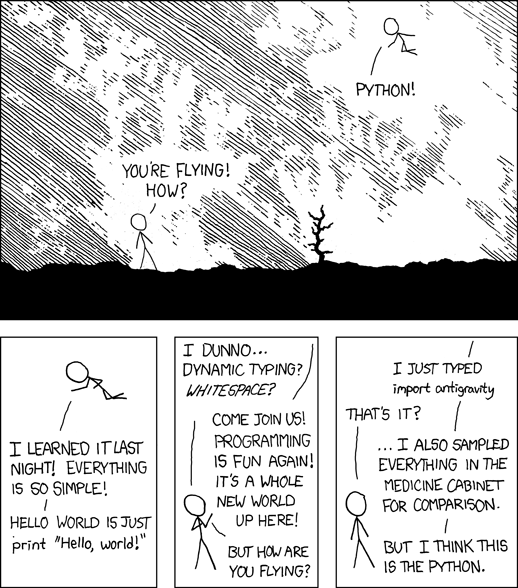# Data manipulation import pickle import pandas as pd import numpy as np # Machine Learning import sklearn import sklearn.ensemble import sklearn.svm from sklearn.cross_validation import train_test_split from sklearn.grid_search import GridSearchCV from sklearn.metrics import confusion_matrix from sklearn.metrics import classification_report # Plot import matplotlib.pyplot as plt import seaborn as sns from pylab import rcParams %matplotlib inline %config InlineBackend.figure_format='retina' rcParams['figure.figsize'] = 8, 5.5 # Utility functions from sklearn.metrics import roc_curve, auc from sklearn.preprocessing import label_binarize # Plot a confusion matrix def plotConfMap(confMat, classes=[], relative=False): width = len(confMat) height = len(confMat) oldParams = rcParams['figure.figsize'] rcParams['figure.figsize'] = width, height fig = plt.figure() plt.clf() plt.grid(False) ax = fig.add_subplot(111) ax.set_aspect(1) if not relative: res = ax.imshow(confMat, cmap='coolwarm', interpolation='nearest') else: res = ax.imshow(confMat, cmap='coolwarm', interpolation='nearest', vmin=0, vmax=100) for x in xrange(width): for y in xrange(height): ax.annotate(str(np.round(confMat[x][y], 1)), xy=(y, x), horizontalalignment='center', verticalalignment='center') fig.colorbar(res) if len(classes) > 0: plt.xticks(range(width), classes) plt.yticks(range(height), classes) rcParams['figure.figsize'] = oldParams return fig # Plot CV scores of a 2D grid search def plotGridResults2D(x, y, x_label, y_label, grid_scores): scores = [s for s in grid_scores] scores = np.array(scores).reshape(len(x), len(y)) plt.figure() plt.imshow(scores, interpolation='nearest', cmap=plt.cm.RdYlGn) plt.xlabel(y_label) plt.ylabel(x_label) plt.colorbar() plt.xticks(np.arange(len(y)), y, rotation=45) plt.yticks(np.arange(len(x)), x) plt.title('Validation accuracy') # Plot CV scores of a 1D "grid" search (a very narrow "grid") def plotGridResults1D(x, x_label, grid_scores): scores = np.array([s for s in grid_scores]) plt.figure() plt.plot(scores) plt.xlabel(x_label) plt.ylabel('Score') plt.xticks(np.arange(len(x)), x, rotation=45) plt.title('Validation accuracy')  Load and split dataset First, let’s load the dataset from our pickle file and split it into 3 sets: • learn for the learning phase, split into: • train for the training (will be used as validation with CV too) • test to evaluate the results • final for the final prediction, the one we will send to Kaggle # Load X_ids, X, y = pickle.load(open('Xy.pkl', 'rb')) y = y.reshape(y.shape) X_ids = np.array(X_ids) # Split learn and final with outcome indices i_final = (y == -1) i_learn = (y > -1) X_ids_final = X_ids[i_final] X_ids_learn = X_ids[i_learn] X_final = X[i_final, :] X_learn = X[i_learn, :] y_learn = y[i_learn] # Split train and test X_train, X_test, y_train, y_test = train_test_split(X_learn, y_learn, test_size=.25)  RBF SVM Having been taught Machine Learning in a large part by a researcher in SVM and kernel methods, my first Machine Learning try on a problem is often to use a SVM. Tuning the hyperparameters To optimize the hyperparameters of my SVM, I’m going to do a double step grid search, first looking at the optimal value on a coarse grid, and then on a more fine grid around a promising area. You can actually do this a lot better, and especially you can accelerate the coarse grid a lot (that’s supposed to be the idea of it being coarse) for example by using a subset of the dataset. Here we might have dropped a lot of non-bots bidders I think. But anyway, that’s how it is… One important thing is of course to think about finding the optimal value considering the AUC a scoring metrics, and not the classification rate. This obviously increase a lot your performance. I chose to use 10 CV because my « bot » class contains really not a lot of values and I don’t want to drop to much of them in the test fold. # Coarse grid C_range = np.r_[np.logspace(-2, 20, 13)] gamma_range = np.r_[np.logspace(-9, 5, 15)] grid = GridSearchCV(sklearn.svm.SVC(C=1.0, kernel='rbf', class_weight='auto', verbose=False, max_iter=60000), {'C' : C_range, 'gamma': gamma_range}, scoring='roc_auc', cv=10, n_jobs=8) grid.fit(X_learn, y_learn) plotGridResults2D(C_range, gamma_range, 'C', 'gamma', grid.grid_scores_) plt.show() # Display result C_best = np.round(np.log10(grid.best_params_['C'])) gamma_best = np.round(np.log10(grid.best_params_['gamma'])) print 'best C coarse:', C_best print 'best gamma coarse:', gamma_best # Fine grid C_range2 = np.r_[np.logspace(C_best - 1.5, C_best + 1.5, 15)] gamma_range2 = np.r_[np.logspace(gamma_best - 1.5, gamma_best + 1.5, 15)] gridFine = GridSearchCV(sklearn.svm.SVC(C=1.0, kernel='rbf', class_weight='auto', verbose=False, max_iter=60000), {'C' : C_range2, 'gamma': gamma_range2}, scoring='roc_auc', cv=10, n_jobs=8) gridFine.fit(X_learn, y_learn) plotGridResults2D(C_range2, gamma_range2, 'C', 'gamma', gridFine.grid_scores_) # Final result bestClf = gridFine.best_estimator_ bestClf.probability = True print bestClf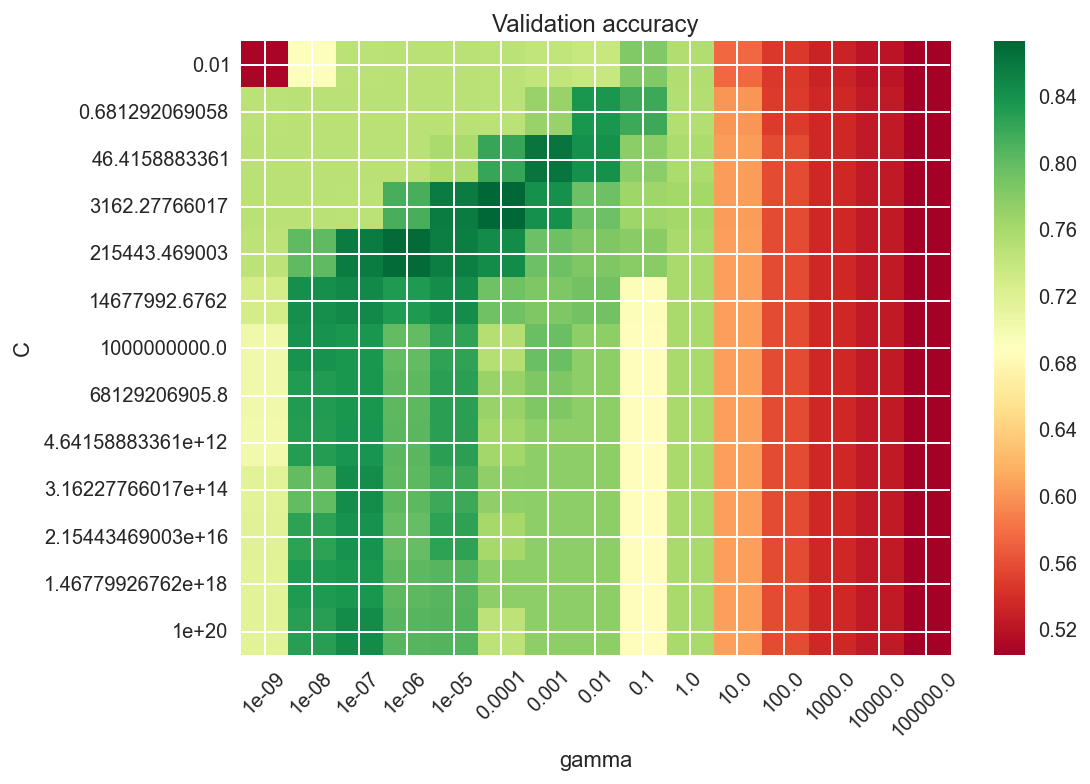best C coarse: 4.0 best gamma coarse: -4.0 SVC(C=517.94746792312128, cache_size=200, class_weight='auto', coef0=0.0, degree=3, gamma=0.00043939705607607906, kernel='rbf', max_iter=60000, probability=True, random_state=None, shrinking=True, tol=0.001, verbose=False)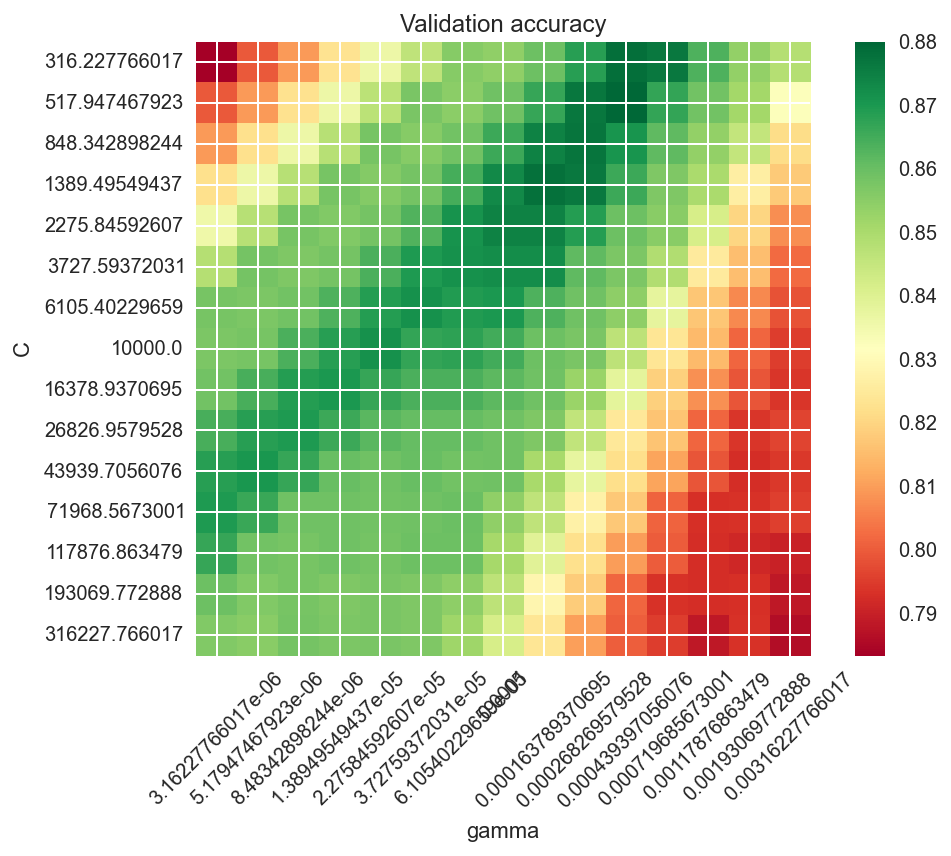Testing the model Now that we have our CV-optimized hyperparameters, let’s learn this classifier on the full train set and evaluate it on the test set. # Fit it bestClf.fit(X_train, y_train) y_pred = bestClf.predict(X_test) # Classif report and conf mat print sklearn.metrics.classification_report(y_test, y_pred) plotConfMap(sklearn.metrics.confusion_matrix(y_test, y_pred)) plt.show() # Predict scores y_score = bestClf.decision_function(X_test) # Plot ROC fpr, tpr, _ = roc_curve(y_test, y_score) roc_auc = auc(fpr, tpr) plt.figure() plt.plot(fpr, tpr, label='ROC curve (area = %0.2f)' % roc_auc) plt.plot([0, 1], [0, 1], 'k--') plt.xlim([0.0, 1.0]) plt.ylim([0.0, 1.05]) plt.xlabel('False Positive Rate') plt.ylabel('True Positive Rate') plt.title('Receiver operating characteristic') plt.legend(loc="lower right") plt.show()   precision recall f1-score support 0.0 0.97 0.82 0.89 464 1.0 0.20 0.62 0.30 32 avg / total 0.92 0.81 0.85 496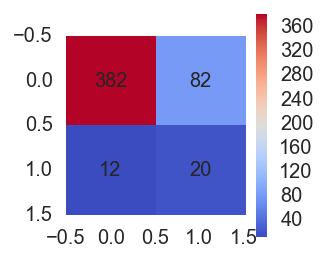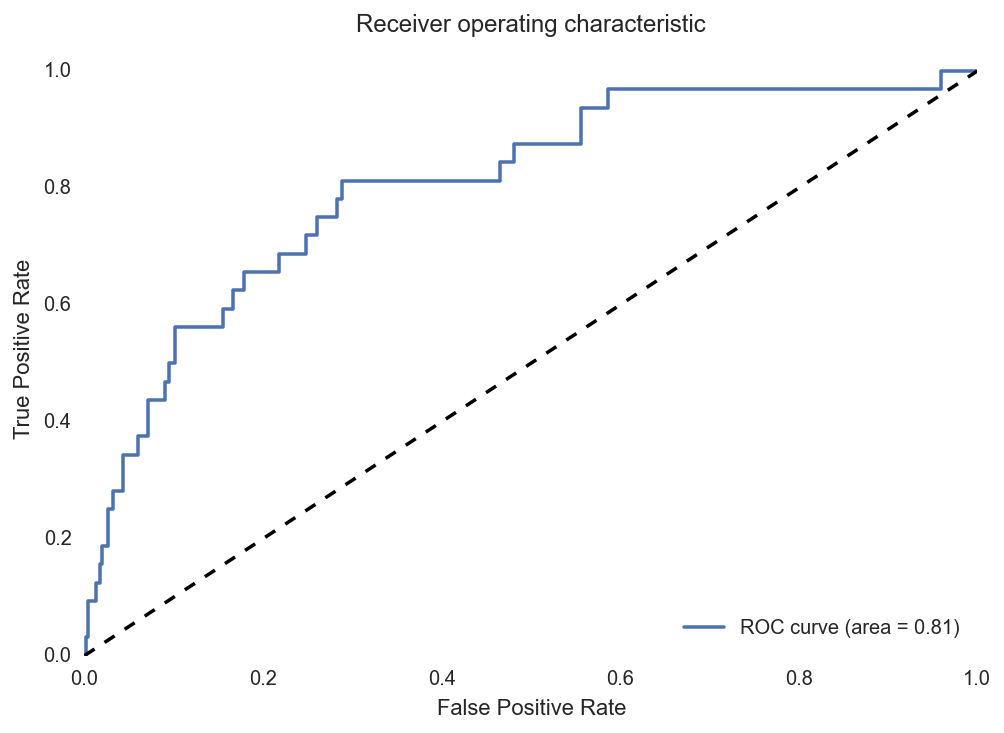Final prediction Ok, now let’s make our final prediction, learing on the full learning set and prediction on the final set. # Fit on learn set and predict final set bestClf.fit(X_learn, y_learn) y_final = bestClf.predict_proba(X_final)[:,1] # Dirty join with test.csv to order them like it was before... # ... now that I look at this I am quite sure that Kaggle don't need them in the right # order so all this is actually pretty stupid. Sorry :) bidders_y_final = pd.DataFrame(np.c_[X_ids[i_final], y_final], columns=['bidder_id', 'prediction']) bidders_y_final[['prediction']] = bidders_y_final[['prediction']].astype(float) bidders_list = pd.read_csv('test.csv', header=0) bidders_list_final = pd.merge(bidders_list[['bidder_id']], bidders_y_final, how='left').fillna(0.0) # Write results to file f = open('predictions_RBF_SVM.csv', 'wb') f.write(bidders_list_final.to_csv(index=False)) f.close()  Linear L1 SVM Because of all the categories we kept from bids (highest frequent category use by a bidder, for a few variables), we have a lot of binary features, so it makes sense to use some L1 regularization. However, scikit-learn’s RBF SVM doesn’t have this feature, and I was too lazy to implement it myself so I tried the linear L1 SVM of scikit-learn. Also I wanted to try linear SVM as well. The code is basically the same as for RBF SVM. Tuning the hyperparameters # Coarse grid C_range = np.r_[np.logspace(-2, 10, 12)] grid = GridSearchCV(sklearn.svm.LinearSVC(C=1.0, penalty='l1', class_weight='auto', dual=False, verbose=False, max_iter=3000), {'C' : C_range}, cv=10, n_jobs=8, scoring='roc_auc') grid.fit(X_learn, y_learn) plotGridResults1D(C_range, 'C', grid.grid_scores_) plt.show() # Results C_best = np.round(np.log10(grid.best_params_['C'])) print 'best C coarse:', C_best # Fine grid C_range2 = np.r_[np.logspace(C_best - 1.5, C_best + 1.5, 15)] gridFine = GridSearchCV(sklearn.svm.LinearSVC(C=1.0, class_weight='auto', dual=False, verbose=False, max_iter=3000), {'C' : C_range2}, cv=10, n_jobs=8, scoring='roc_auc') gridFine.fit(X_learn, y_learn) plotGridResults1D(C_range2, 'C', gridFine.grid_scores_) # Final results bestClf = gridFine.best_estimator_ bestClf.probability = True print bestClf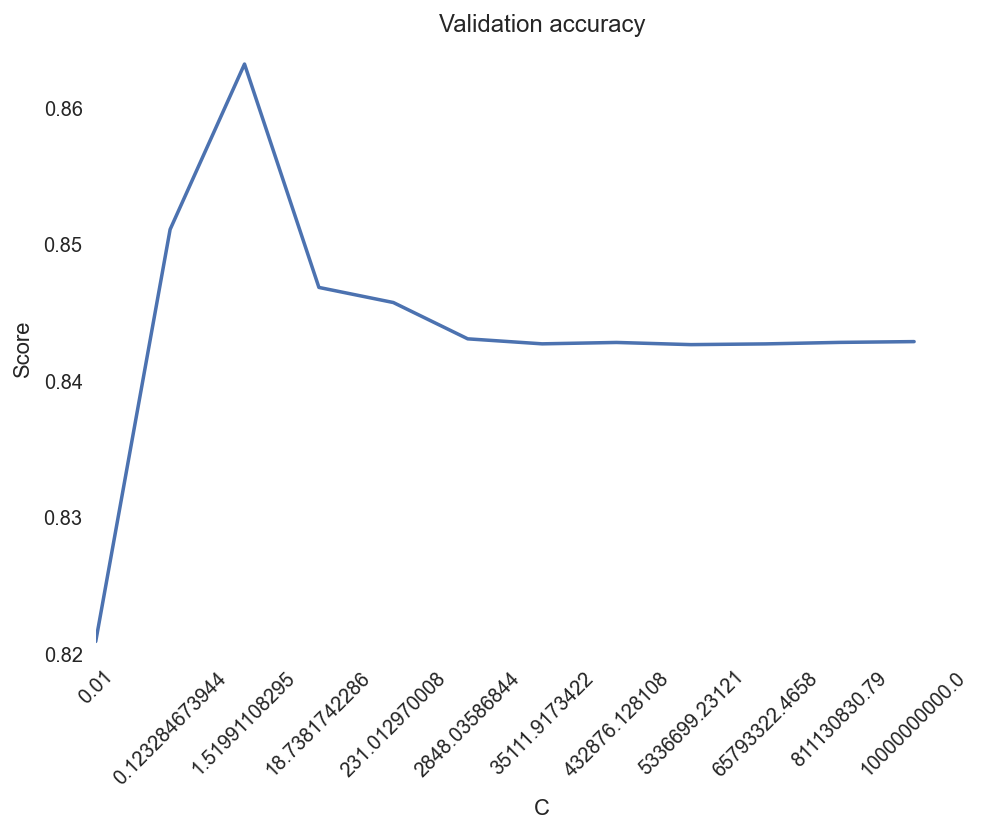best C coarse: 0.0 LinearSVC(C=0.13894954943731375, class_weight='auto', dual=False, fit_intercept=True, intercept_scaling=1, loss='squared_hinge', max_iter=3000, multi_class='ovr', penalty='l2', random_state=None, tol=0.0001, verbose=False)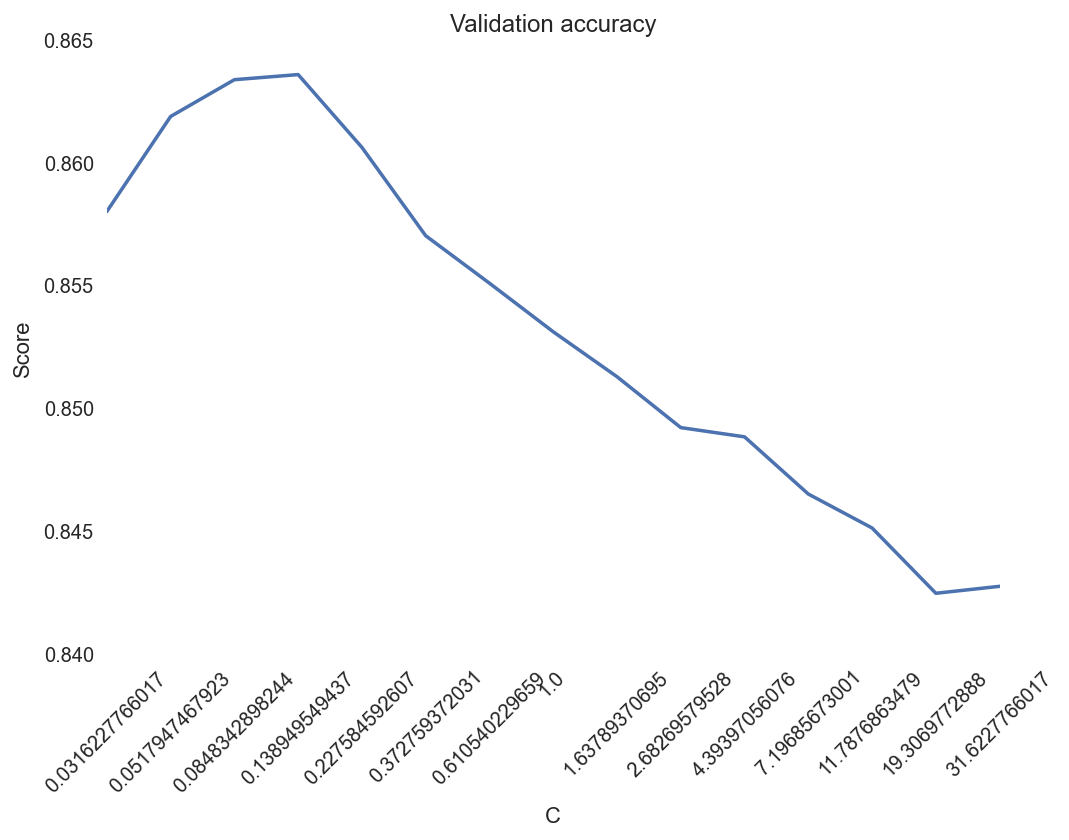Testing the model # Fit on train bestClf.fit(X_train, y_train) y_pred = bestClf.predict(X_test) # Classification report print sklearn.metrics.classification_report(y_test, y_pred) plotConfMap(sklearn.metrics.confusion_matrix(y_test, y_pred)) plt.show() # Predict y_score = bestClf.decision_function(X_test) # Plot ROC fpr, tpr, _ = roc_curve(y_test, y_score) roc_auc = auc(fpr, tpr) plt.figure() plt.plot(fpr, tpr, label='ROC curve (area = %0.2f)' % roc_auc) plt.plot([0, 1], [0, 1], 'k--') plt.xlim([0.0, 1.0]) plt.ylim([0.0, 1.05]) plt.xlabel('False Positive Rate') plt.ylabel('True Positive Rate') plt.title('Receiver operating characteristic') plt.legend(loc="lower right") plt.show()   precision recall f1-score support 0.0 0.98 0.80 0.88 464 1.0 0.19 0.72 0.31 32 avg / total 0.93 0.79 0.84 496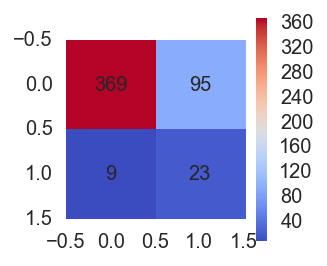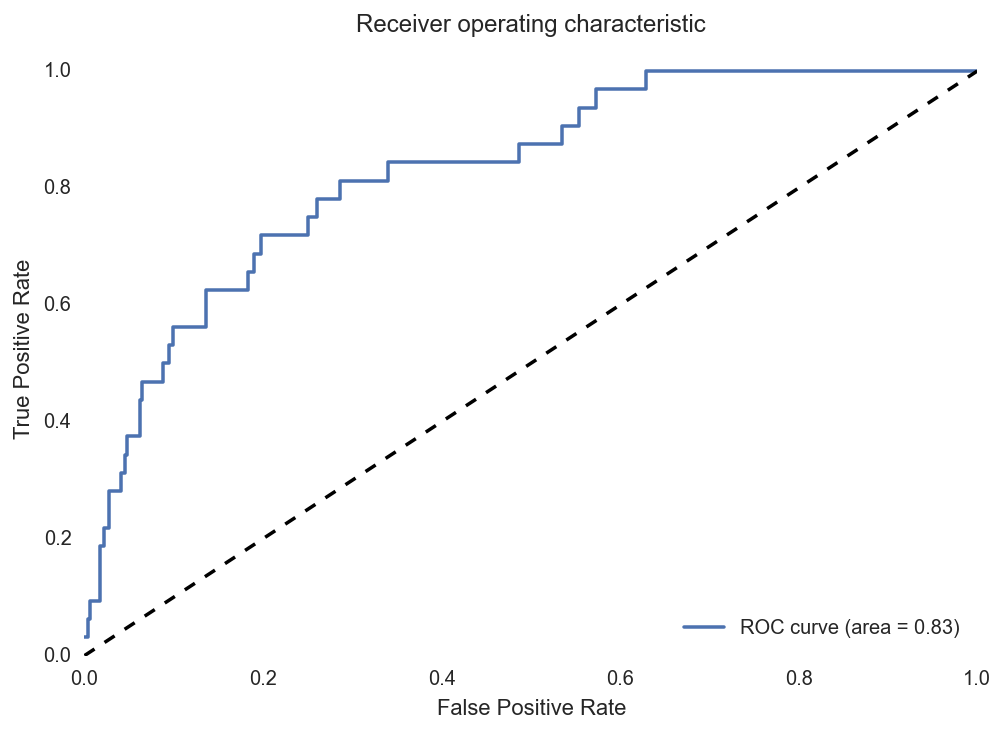Final prediction # Fit on learn bestClf.fit(X_learn, y_learn) y_final = bestClf.predict(X_final) # Reorder prediction bidders_y_final = pd.DataFrame(np.c_[X_ids[i_final], y_final], columns=['bidder_id', 'prediction']) bidders_y_final[['prediction']] = bidders_y_final[['prediction']].astype(float) bidders_list = pd.read_csv('test.csv', header=0) bidders_list_final = pd.merge(bidders_list[['bidder_id']], bidders_y_final, how='left').fillna(0.0) # Save to file f = open('predictions_Lin_SVM_L1.csv', 'wb') f.write(bidders_list_final.to_csv(index=False)) f.close()  Random forest Also a classifier well known to be great is the random forest. The problem is that it can have a lot of hyperparameters to tune. Basically all the parameters in the trees plus the ensemble settings such as number of estimators, but also random feature subspace size if you want, etc. I chose to only CV grid search the number of estimators and the max depth of the tree. The idea of this code is again very close to the one of SVMs. But in this case I only used one grid search. If I wanted a perfect there would definitely be things to factorize between those different classifiers (and scikit-learn is actually very good for this with their consistant interfaces across classifiers). Tuning the hyperparameters # Coarse grid HP_range = np.array([1, 2, 3, 4, 5, 6, 7, 8, 9, 10, 12, 15, 18, 20, 25, 30, 50, 100]) HP2_range = np.array([11, 21, 35, 51, 75, 101, 151, 201, 251, 301, 351, 401, 451, 501]) grid = GridSearchCV(sklearn.ensemble.RandomForestClassifier(n_estimators=300, max_depth=None, max_features='auto', class_weight='auto'), {'max_depth' : HP_range, 'n_estimators' : HP2_range}, cv=sklearn.cross_validation.StratifiedKFold(y_learn, 5), n_jobs=8, scoring='roc_auc') grid.fit(X_learn, y_learn) plotGridResults2D(HP_range, HP2_range, 'max depth', 'n estimators', grid.grid_scores_) plt.show() # Final res bestClf = grid.best_estimator_ print bestClf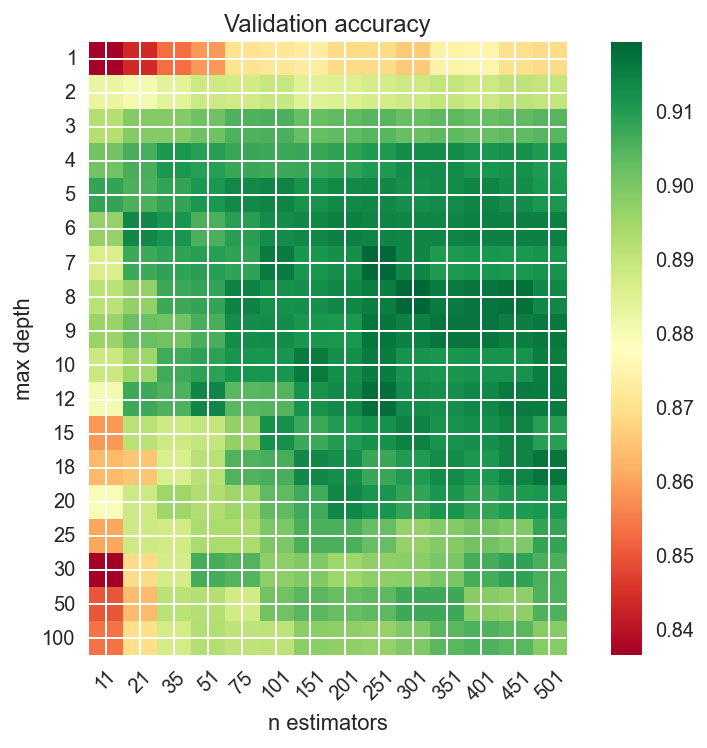RandomForestClassifier(bootstrap=True, class_weight='auto', criterion='gini', max_depth=8, max_features='auto', max_leaf_nodes=None, min_samples_leaf=1, min_samples_split=2, min_weight_fraction_leaf=0.0, n_estimators=301, n_jobs=1, oob_score=False, random_state=None, verbose=0, warm_start=False)  Testing the model # Ok, I actally want to choose these paramters myself! I'm god here, I do what I want! bestClf = sklearn.ensemble.RandomForestClassifier(max_depth=20, n_estimators=301, max_features='auto', class_weight='auto') # Learn on train for test bestClf.fit(X_train, y_train) y_pred = bestClf.predict(X_test) # Classification report print sklearn.metrics.classification_report(y_test, y_pred) plotConfMap(sklearn.metrics.confusion_matrix(y_test, y_pred)) plt.show() # Predict scores y_score = bestClf.predict_proba(X_test)[:,1] # ROC fpr, tpr, _ = roc_curve(y_test, y_score) roc_auc = auc(fpr, tpr) plt.figure() plt.plot(fpr, tpr, label='ROC curve (area = %0.2f)' % roc_auc) plt.plot([0, 1], [0, 1], 'k--') plt.xlim([0.0, 1.0]) plt.ylim([0.0, 1.05]) plt.xlabel('False Positive Rate') plt.ylabel('True Positive Rate') plt.title('Receiver operating characteristic') plt.legend(loc="lower right") plt.show()   precision recall f1-score support 0.0 0.94 1.00 0.97 464 1.0 0.83 0.16 0.26 32 avg / total 0.94 0.94 0.93 496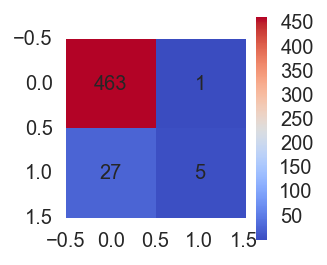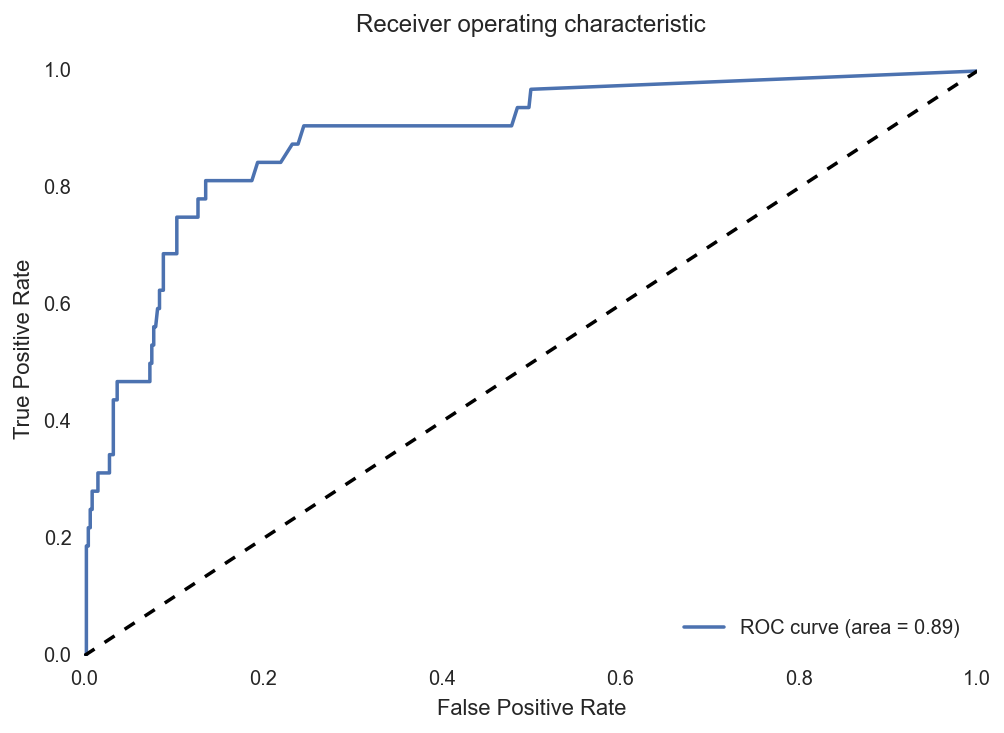bestClf.fit(X_learn, y_learn) y_final = bestClf.predict_proba(X_final)[:,1] bidders_y_final = pd.DataFrame(np.c_[X_ids[i_final], y_final], columns=['bidder_id', 'prediction']) bidders_y_final[['prediction']] = bidders_y_final[['prediction']].astype(float) bidders_list = pd.read_csv('test.csv', header=0) bidders_list_final = pd.merge(bidders_list[['bidder_id']], bidders_y_final, how='left').fillna(0.0) f = open('predictions_RF_2.0.csv', 'wb') f.write(bidders_list_final.to_csv(index=False)) f.close()  AdaBoost Ok I also tried AdaBoost which is also a nice ensemble technique but it didn’t gave particularly better results than random forest. I think you got the idea of my code so I spare you the code for AdaBoost… Conclusion I finally submitted predictions of my random forest for evaluation on the private leaderboard. I think this competition was more about feature engeneering than the pure Machine Learning part. There are a lot of things I could have improved in my work. First, I could have used more complex features. Some good examples can be found for example on Kaggle’s forum of the competition « share your secret sauce » thread, or other similar threads. I think more complex time series analysis could have been done on bids times. I did not tried to clean the dataset, but I think that removing some wierd points from the training dataset might have been a good thing. Also I think there might be interesting ways to deal with the imbalance of the dataset (other than class weighting that I did of course), but I didn’t found time to dig into this. Finally regarding the pure Machine Learning part, using ensemble of different classifiers like some people did could also have improved the results. Anyway, I am quite surprised of the good results I got with so simple features and model compared to what some other people did. LogAnalysis : outil web open-source de Business Intelligence gégraphiqueContexte En ASI (Architecture des Systèmes d’Information), à l’INSA de Rouen, le second semestre de 4ème année et le premier semestre de 5ème année sont l’occasion pour les étudiants de travailler sur un Projet INSA Certifié (PIC), un projet industriel type R&D commandé par une entreprise et réalisé par les étudiants. 5 sujets sont réalisés par 6 équipes de 7 à 9 étudiants durant 1 an scolaire à raison de 25 à 28h par semaine et par personne. Pour être exact, une des équipes n’existe que pendant le premier semestre de PIC et joue le rôle de sous-traitant pour une autre équipe. Cette équipe disparaît au second semestre de PIC pour permettre aux étudiants de partir à l’étranger. Sujet Le sujet sur lequel mon équipe à travaillé consiste à développer un outil open-source permettant de faire de Business Intelligence géographique, et surtout, de la rendre naturelle à l’utilisateur, accessible sans connaissance de la théorie de la « GeoBI ». Ce sujet nous a été proposé par le Centre de Recherche Publique Henri Tudor, basé au Luxembourg, maintenant nommé Luxembourg Institute for Science and Technology (LIST). L’équipe sous-traitante a travaillé avec nous sur ce sujet. La Business Intelligence En très résumé, la Business Intelligence (BI) ou informatique décisionnelle, c’est le fait de pouvoir analyser des grandes quantités de données généralement structurées selon un grand nombre de dimensions. Par exemple, le chiffre d’affaire en fonction des dimensions temps, espace, client, secteur d’activité, produit, etc. La Business Intelligence à pour but de permettre d’analyser ces masses de données structurées afin d’en faire émerger une décision éclairée. On peut décomposer la Business Intelligence entre 3 grandes étapes : 1. Collecte des données afin de constituer une base de données structurées 2. Sélection et traitement des données 3. Affichage des données à l’utilisateur Collecte des données Cette étape préliminaire à la Business Intelligence n’est pas traitée dans le cadre du PIC car elle est spécifique à chaque entité désirant faire de la Business Intelligence. Son but est de collecter les données venant de multiples sources afin de constituer une base de données la plus précis et complète possible. Ces données sont alors stockées dans des data warehouses et datamarts. Cela constitue alors des bases de données OLAP. Sélection et traitement des données La première étape d’une analyse de BI est la sélection des données intéressantes à l’étude que l’utilisateur veut mener (ex : sélection des données de la France en 2014 uniquement). C’est le slice OLAP. Le traitement de données le plus courant est ensuite de choisir comment agréger les données afin d’en obtenir des résultats pertinents (ex : agrégation par mois et par département). C’est le dice OLAP. C’est – de façon réductrice bien sûr – le principe de l’OLAP : limiter le champ de données et l’agréger aux niveaux les plus pertinents. Affichage des données La deuxième étape d’une analyse de BI est l’affichage des données à l’utilisateur. L’affichage le plus simple est l’affichage d’un tableau. Ce n’est cependant pas la façon la plus simple pour réussir à interpréter les données. On introduit alors des graphiques, des cartes choroplèthes, etc.Résultats d’une analyse de BI – Crédits : http://apandre.wordpress.com/ Objectifs du projet & démonstration Ce projet avait donc pour but de développer une interface web permettant de réaliser des analyses de BI géographique en s’intégrant à GeoNode, plateforme qui offre déjà des outils de gestion de données géographiques. Le tout a été développé sous licence Open Source (GPLv3) est est disponible sur GitHub. Les objectifs du projets étaient les suivants : • Traitement et récupération des données depuis la base de données OLAP GeoMondrian • Affichage des résultats sous la forme de divers graphiques : carte choroplèthe, diagramme en secteur (pie chart), en barres (bar chart), à bulles (bubble chart), tableau, nuage de mots (word cloud) • Interaction avec les graphiques permettant a l’utilisateur d’interroger la base de données OLAP et de réaliser son analyse de BI de façon intuitive et naturelle : • Filtrage dynamique (slice OLAP) au clic ; • Zoom dimensionnel (drill-down et roll-up OLAP modifiant donc à la fois le slice et le dice) au zoom molette. Par exemple, un drill-down sur la France affichera les régions de France. Différentes version du drill-down sont possible : simple (sur 1 élément), multiple (plusieurs éléments), partiel (drill-down sur un élément tout en conservant les éléments du niveau supérieur permettant de comparer des données de différents niveaux, par exemples les régions de France et les autres pays d’Europe) Voici une démonstration rapide de l’application permettant d’avoir un aperçu rapide des objectifs : Architecture Architecture globale Le diagramme ci-dessous décrit l’architecture globale de la solution développée :On y retrouve 4 composants : • L’interface web permettant la visualisation des données. Elle se compose de plusieurs éléments : • analytics.js, le package développé pour gérer l’interface • QueryAPI, un proxy des interfaces de Mandoline, l’API SOLAP • crossfilter, une sorte de petit serveur OLAP côté client • crossfilter server, une librairie développée pour permettre de réaliser les mêmes operations que crossfilter, mais déléguant les calculs OLAP au serveur via une API • d3.js, une librairie utilisée pour les rendus SVG • dc.js, une version modifiée du dc.js original pour réaliser des rendus de graphiques interactifs de données dimensionnelles • Une application web en Python, analytics, délivrant l’interface web, étendant l’application web existante GeoNode • Une API SOLAP en Java nommée Mandoline, et sa dépendance olap4j, nous permettant d’interroger GeoMondrian simplement en JSON • La base de données OLAP avec le serveur SOLAP GeoMondrian récupérant les données depuis une base PostgreSQL, réalisant le traitement des données analytics.js Le package analytics.js représente la majeure partie du travail réalisé sur ce projet. Il est composé de divers « namespaces », chacun contenant diverses et « classes », ces appellation étant utilisé pour la compréhension du propos puisqu’étant en JavaScript, ces types d’éléments n’existent pas et sont dans notre cas des objets. Voici l’architecture du package :On y trouve les namespaces : • analytics.query qui simplifie l’interrogation de l’API SOLAP via QueryAPI en offrant des fonctions spécialisées • analytics.data qui offre des types de données permettant de représenter les éléments OLAP, et qui stocke les données reçues • analytics.state qui stocke et offre des fonctions permettant de modifier l’état OLAP de l’analyse en cours (pour simplifier, quels éléments de quels niveaux sont en cours d’étude) • analytics.display gère l’interface (où est chaque graphique et qu’affiche-t-il) et les interactions avec celle-ci • analytics.charts offre des classes pour chaque type de graphique qui peuvent être instanciées pour chaque nouveau graphique Cross-filtering and crossfilter-server.js Une des problématiques majeures du projet est de fournir un filtrage croisé efficace sur l’interface. On peut voir cette fonctionnalité dans la vidéo de démonstration. En effet, ce filtrage est très pratique pour l’utilisateur car elle lui permet de voir simplement comment les données se distribuent parmi les divers éléments d’une dimension et d’explorer le cube OLAP. Cette fonction de filtrage est donc fortement sollicitée et se doit d’être particulièrement efficace tout en supportant de fort volumes de données. Il y a en fait deux solutions permettant de mettre en oeuvre cette fonctionnalité, représentées sur ce schéma :On voit sur ce graphique le cube OLAP à gauche qui stocke les données, et à droite des graphiques que l’utilisateur veut visualiser. Première solution : Crossfilter La première possibilité est de demander au serveur OLAP, GeoMondrian, de calculer un cube de données plus petit contenant ce que l’on est en train d’étudier. Sur le diagramme, ce cube intermédiaire est situé au centre et contient les données par région de France, années et mode de transport. Ce cube temporaire est téléchargé dans le navigateur de l’utilisateur et fourni a crossfilter. Crossfilter pourra ensuite calculer les projections de ce cube sur chaque dimension, et ces projections seront utilisées par dc.js pour réaliser les rendus. Dans cette solution, l’interface sera très réactive au filtrage, car crossfilter possède déjà toutes les données dont il a besoin pour calculer les projections en tenant compte des filtres, et pourra réaliser les calculs en moins d’une seconde. Cependant, le défaut de cette solution est le nombre de valeurs dans le cube, qui est le produit du nombre d’éléments étudiés dans chaque dimension (par exemple pour 6 régions, 2 ans et 3 modes de transport on a 6 × 2 × 3 = 36 valeurs), et cette taille augmente donc exponentiellement. On ne pourra donc supporter que des volumes raisonnables car le cube finira par devenir trop lourd pour être téléchargé en un temps raisonnable. Seconde solution : Crossfilter Server L’alternative consiste à demander à GeoMondiran de calculer directement les projections du cube OLAP, après filtrage, sur chaque dimension étudiée sans calculer le cube temporaire. Dans ce cas, la taille des données chargés est la somme du nombre d’éléments étudiés dans chaque dimension (par exemple pour 6 régions, 2 ans et 3 modes de transport on a 6 + 2 + 3 = 11 valeurs), et cette taille augmente linéairement. On sera donc capable de supporter des volumes importants car on charge bien moins de données (tant que GeoMondrian supporte ces volumes bien sûr). Cependant, l’inconvenient de cette solution est que quand l’utilisateur change de filtres, on doit demander à nouveau à GeoMondrian les projections sur chaque dimension en prenant en compte les nouveaux filtres. Cela ralenti donc le temps de réaction lors du filtrage car on doit interroger le serveur là ou crossfilter faisait les calculs localement. Un autre problème de cette solution est que notre librairie d’affichage, dc.js, est dépendante de crossfilter en tant que modèle de données, on ne peut pas lui donner directement des données. Pour répondre à ce problème et rendre cette alternative possible, nous avons développé une petite librairie nommée Crossfilter Server. Cette librairie a presque les même interfaces d’I/O que Crossfilter (seule l’initialisation change). Crossfilter Server va donc reproduire le fonctionnement de Crossfilter sans posséder les données (le cube intermédiaire), il va déléguer les calculs à une API qui va, dans notre cas, interroger GeoMondrian. Ainsi, nous pouvons utiliser dc.js indifféremment avec Crossfilter ou Crossfilter Server. Récapitulatif & solution Pour résumé, nous avons 2 solutions :Crossfilter •\mathcal{O}(n^p)\left(\prod_{i \in \mathcal{D}} n_i\right)\Rightarrow$Volumes raisonnables • Filtrage & agrégats côté client rapide (< 10 ms)$\Rightarrow$Excellente réactivité Crossfilter Server •$\mathcal{O}(n\times p)\left(\sum_{i \in \mathcal{D}} n_i\right)\Rightarrow$Volumes importants • Filtrage & agrégats côté serveur, rechargement ($\approx$1s)$\Rightarrow$Réactivité réduite Notre solution définitive consiste a utiliser ces deux solutions, en utilisant l’une ou l’autre selon le volume de données en cours d’étude, en passant dynamiquement de l’une à l’autre selon les besoin. Conclusion Cet article est bien sûr un résumé rapide d’un projet conséquent. Si vous voulez davantage d’informations, vous pouvez consulter l documentation du projet disponible sur le wiki du dépôt GitHub ou jeter un oeil au code des composants listés précédemment. Si vous voulez, vous pouvez même contribuer à ce projet en faisant une pull-request ! 17 équations qui ont changé le mondeCet article est uniquement disponible en anglais pour l’instant. I just finished the excellent book Seventeen Equations That Changed The World by Ian Stewart! From the title, you might think that this book will describe a few equations. It’s actually a lot more. Each equation is actually just a pretext to present a period of time and / or a field of mathematics related the equation. Don’t expect any important calculus in this book, it’s all about historical scientific context and usage. And it was actually a good thing for me. I knew and had used almost all the equations equations presented in this book, but there is actually not a lot of things that I knew from this book. The equations presented in the book are: Title Formula Author & Date Pythagoras Theorem$a^2+b^2=c^2$Pythagoras (530 BC) Logarithms$\log xy=\log x+\log y$John Napier (1610) Calculus$\frac{df}{dt}=\lim\limits_{h \to 0} \frac{f(t+h)-f(t)}{h}$Newton (1668) Law of Gravity$F=G\frac{m_1 m_2}{d^2}$Newton (1687) Imaginary Number$i^2=-1$Euler (1750) Euler’s Formula for Polyhedra$V-E+F=2$Euler (1751) Normal Distribution$\Phi(x) = \frac{1}{{\sigma \sqrt {2\pi } }}e^\frac {( x – \mu)^2 } {2\sigma ^2 }$C.F. Gauss (1810) Wave Equation$\frac{\partial ^2 u}{\partial t^2}=c^2\frac{\partial ^2 u}{\partial x^2}$J. d’Alembert (1746) Fourier Transform$f(\omega )=\int_{-\infty }^{+\infty }f(x) e^{-2\pi ix\omega }dx$J. Fourier (1822) Navier-Stokes Equation$\rho \left(\frac{\partial v}{\partial t}+v\cdot\triangledown v \right) = -\triangledown p + \triangledown\cdot T+f$C. Navier, G. Stoker (1845) Maxwell’s Equations$\triangledown\cdot E=0\triangledown\cdot H=0\triangledown \times E=-\frac{1}{c} \frac{\partial H}{\partial t}\triangledown \times H=\frac{1}{c}\frac{\partial E}{\partial t}$James Clerk Maxwell (1865) Second Law of Thermodynamics$dS\geq 0$Ludwig Boltzmann (1874) Relativity$E=mc^2$Albert Einstein (1905) Schrödinger’s Equation$i\hslash \frac{\partial }{\partial t} \psi=H\psi$Erwin Schrödinger (1927) Information Theory$H=-\sum p(x)\log p(x)$Claude Shannon (1949) Chaos Theory$x_{t+1}=kx_t(1-x_t)$Robert May (1975) Black-Scholes Equation$\frac{\sigma ^2}{2}S^2\frac{\partial ^2V}{\partial S^2}+rS\frac{\partial V}{\partial S}+\frac{\partial V}{\partial t}-rV=0$F. Black, M. Scholes (1990) As I said, it’s actually a lot more than about the equations. For example, the first chapter about Pythagoras theorem will of course present the history of this well known formula and some ideas about the proof of it. But a big part of the chapter is about the history of mathematical symbolism and how we went from the original Pythagoras theorem, that was a sentence, to the current notation$a^2 + b^2 = c^2\$, the difference between math and science knowledge at that time and math nowadays (for example about the shape of Earth). The chapter will also evoke all the mathematical field of geometry, mentioning Elements by Euclid, the definition of trigonometry and its usage for map making and astronomy for example, the existence of non-Euclidean geometry, and even more!

I would highly recommend you this book if you like science and/or engineering, and even if you’re not a particularly a big fan of math.

Si vous voulez un aperçu de ce livre, vous pouvez lire des extraits sur Google Books pour la version française ou la version anglaise ou Amazon pour la version anglaise par exemple.